# What is the angle of rotation on a corresponding element in physical space if the angle...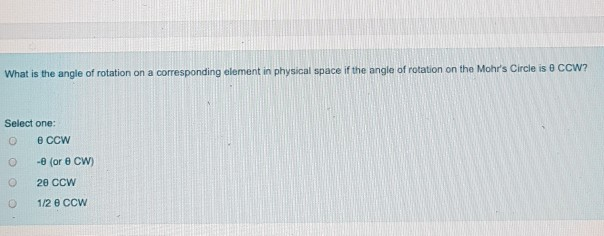What is the angle of rotation on a corresponding element in physical space if the angle of rotation on the Mohr's Circle is 0 CCW? Select one: O CCW - (or e CW 28 CCW 112 CCW

Solution:

Explanation:

For an angle 'x' in the space in counterclockwise direction, the same angle is represented as 2x in Mohr Circle. So if the angle in the Mohr Circle will be x then the angle in the space will be (1/2 x).

So, for angle theta in Mohr Circle in CCW direction , the angle of rotation on the corresponding element in physical space will be (1/2 theta) CCW.

So , last option is correct.

#### Earn Coin

Coins can be redeemed for fabulous gifts.

Similar Homework Help Questions
• ### 1. For the element shown, use Mohr's circle to find the principal stresses and the rotation...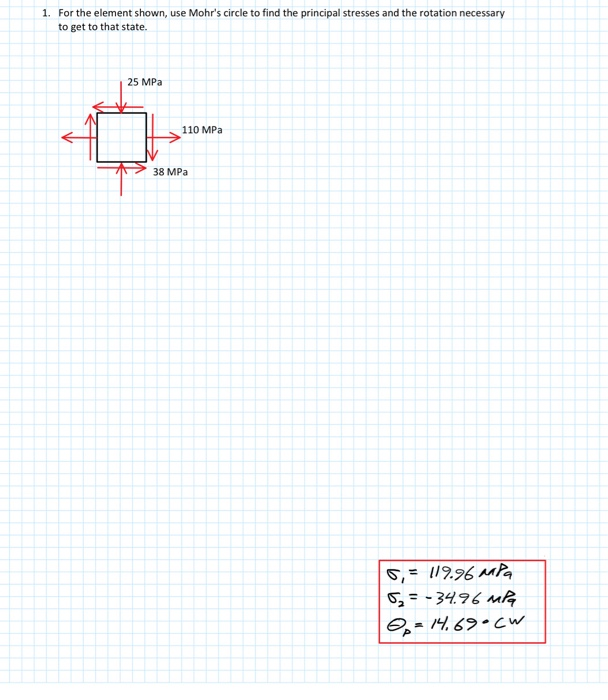1. For the element shown, use Mohr's circle to find the principal stresses and the rotation necessary to get to that state. 25 MPa 110 MPa 38 MPa 5,= 119.96 MPa 52 = - 34.96 MPa 0,- 14.69.cw

• ### Calibri rWrap Text General - 10 TT 19 Copy -A A == R Normal Conditional format...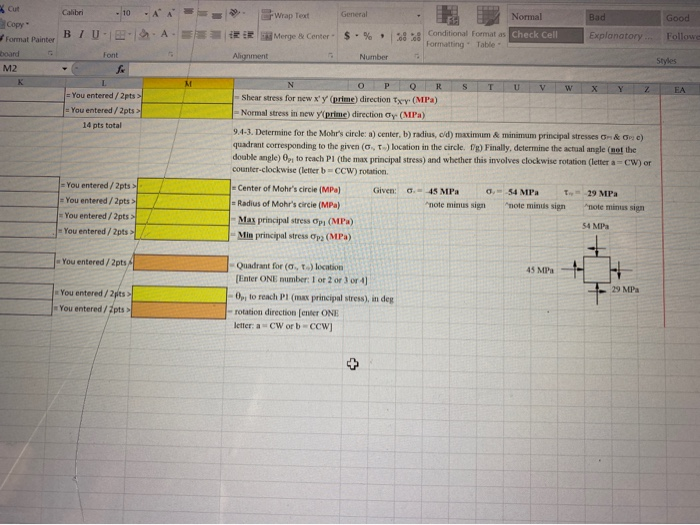Calibri rWrap Text General - 10 TT 19 Copy -A A == R Normal Conditional format as Check Cell Formatting Table Bad Explanatory Good Follow B U Merge Center S. Alignment Number Styles M2 - S T U V W X Y Z E A = you entered/2pts > = you entered/2pts > O P Q R Shear stress for new x'y (prime direction Txy (MPa) Normal stress in new y(prime) direction dy (MPa) 14 pts total - You entered/2pts...

• ### 3. For each of the plane stress states listed below draw a Mohr's Circle diagram properly...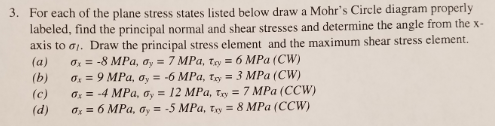3. For each of the plane stress states listed below draw a Mohr's Circle diagram properly labeled, find the principal normal and shear stresses and determine the angle from the X- axis to di. Draw the principal stress element and the maximum shear stress element. (a) 0 = -8 MPa, ay = 7 MPa, Try = 6 MPa (CW) (b) 0 = 9 MPa, y = -6 MPa, Try = 3 MPa (CW) (c) 0:= -4 MPa, y = 12...

• ### Please draw each point PROBLEM 4 (25%) Given the following state of stress, 20 ksi 16...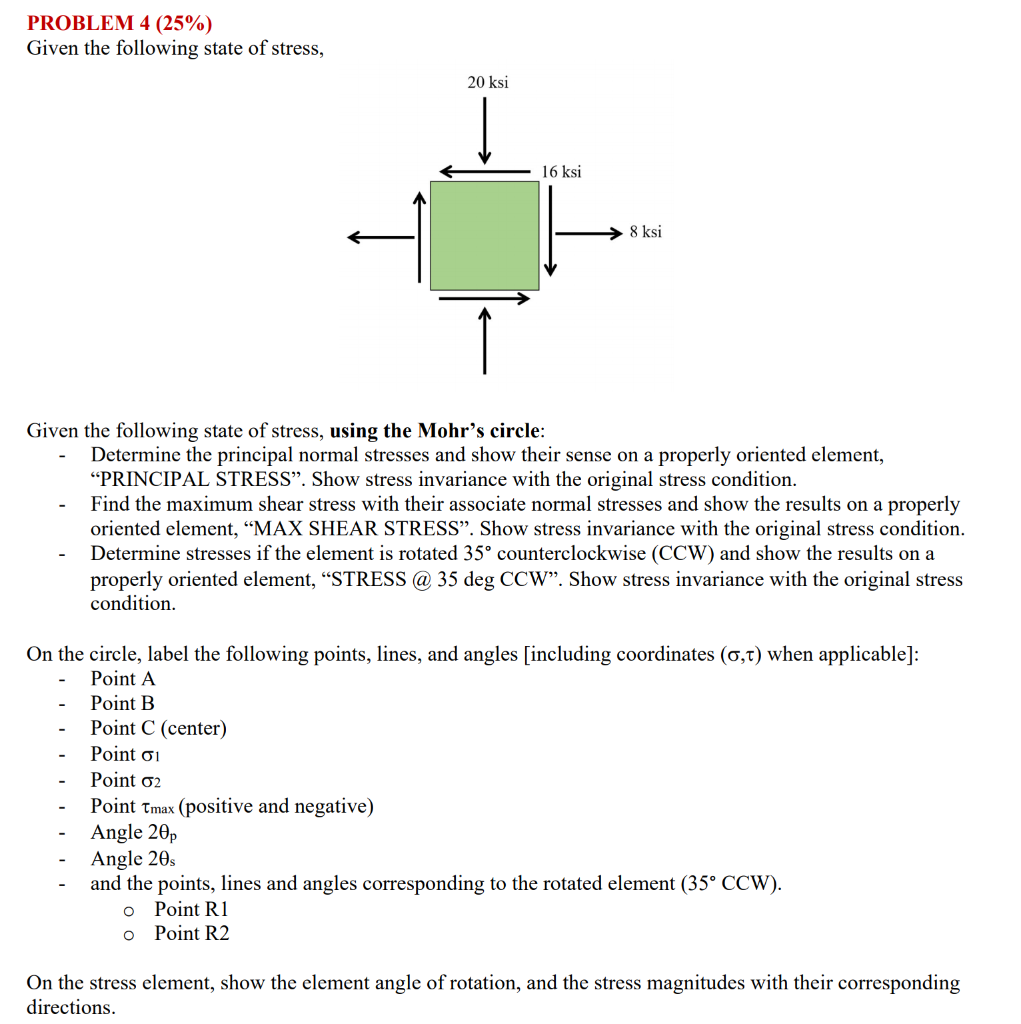Please draw each point PROBLEM 4 (25%) Given the following state of stress, 20 ksi 16 ksi 8 ksi Given the following state of stress, using the Mohr's circle: Determine the principal normal stresses and show their sense on a properly oriented element, “PRINCIPAL STRESS”. Show stress invariance with the original stress condition. Find the maximum shear stress with their associate normal stresses and show the results on a properly oriented element, "MAX SHEAR STRESS”. Show stress invariance with the...

• ### First recall that the matrix corresponding to a rotation by an angle @ is given by...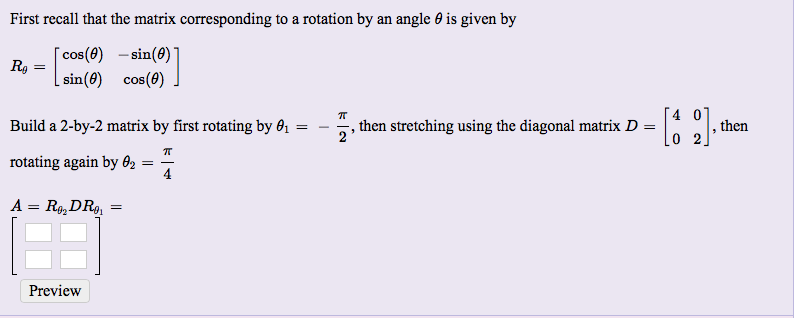First recall that the matrix corresponding to a rotation by an angle @ is given by Ri= cos(0) -sin(0) 1 sin(0) cos(0) ] 0 as wing the diagonal maria D = ( 3 9).son , then 10 21 Build a 2-by-2 matrix by first rotating by 01 = -6, then stretching using the diagonal matrix D= rotating again by 62 = Í . A = Ry, DR, = Preview

• ### ロ ラ| Week 10-ET-2220.101-LE( | [ HW 20 Fall 2018.pdf | LTX v ← → Ο...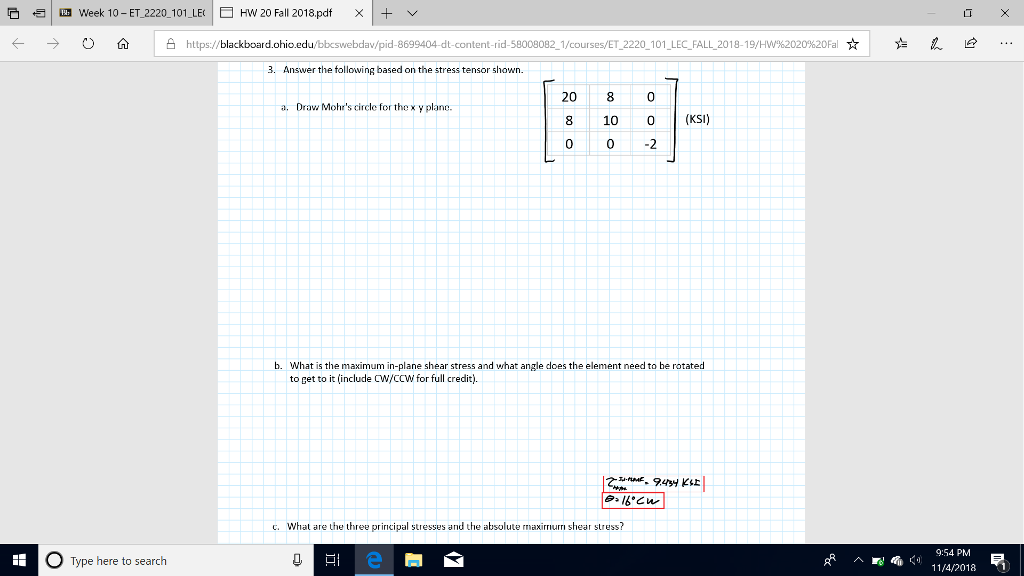ロ ラ| Week 10-ET-2220.101-LE( | [ HW 20 Fall 2018.pdf | LTX v ← → Ο 仚 https //blackboard or o edu bbcswebdav/pid-8699404-dt-content rid 58008082 1/courses ET 2220 101 LEC FALL 2018 19/HM%2020%20Fal ☆ 左 这 四 3. Answer the tollo based on the stress tensor shown. 20 8 0 8 10 0 0-2 a. Draw Mohr's circle for thex yplan 8 10 0(KSI) What is the maximum in-plane shear stress and what angle does the element need to be...

• ### The state of stress on a certain elementos presented by Morse 90 M 60 50. 4.7...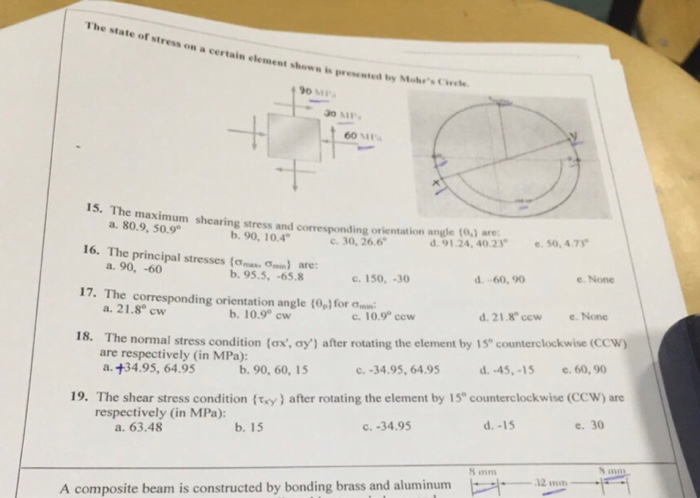The state of stress on a certain elementos presented by Morse 90 M 60 50. 4.7 None 15. The maximum sharing stress and corresponding orientation angle (0.00 a. 80.9, 50.9° b. 90, 10.4 c. 30, 26.6 16. The principal stresses (...) are: a. 90, 60 b. 95.5, -65.8 c. 150, -30 d. 60, 90 17. The corresponding orientation angle (0,} for a. 21.8 cw b. 10.9° cw e. 10.9 cew d. 21.8 cew e. None 18. The normal stress condition...

• ### Given the stress element below: 1. Draw Mohr Circle for the stress element shown below. Use...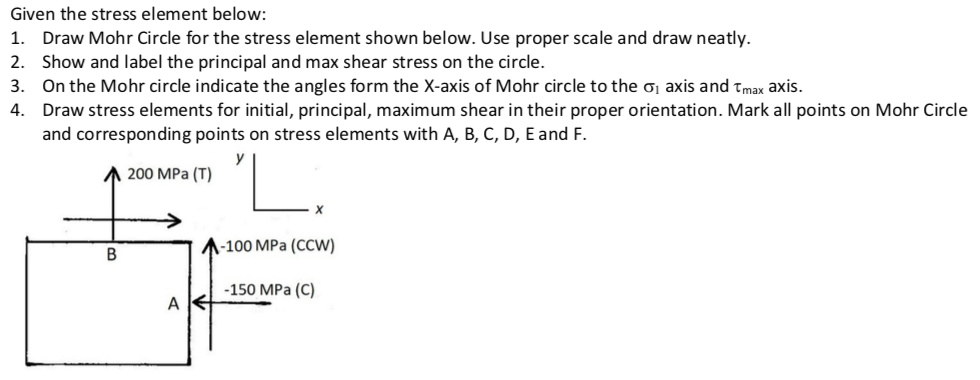Given the stress element below: 1. Draw Mohr Circle for the stress element shown below. Use proper scale and draw neatly. 2. Show and label the principal and max shear stress on the circle. 3. On the Mohr circle indicate the angles form the X-axis of Mohr circle to the o axis and Tmax axis. 4. Draw stress elements for initial, principal, maximum shear in their proper orientation. Mark all points on Mohr Circle and corresponding points on stress elements...

• ### 424· The complex electric field of a uniform 4.26. In a source-free, free-space region, the plane...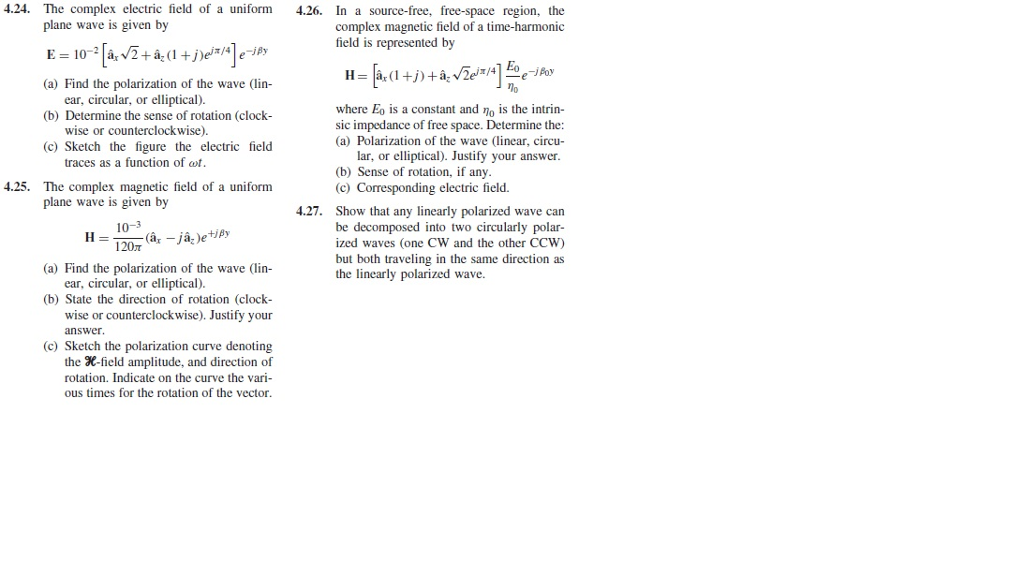424· The complex electric field of a uniform 4.26. In a source-free, free-space region, the plane wave is given by complex magnetic field of a time-harmonic field is represented by (a) Find the polarization of the wave (lin- ear, circular, or elliptical) (b) Determine the sense of rotation (clock wise or counterclockwise). (c) Sketch the figure the electric field where EO is a constant and Po is the intrin- sic impedance of free space. Determine the: (a) Polarization of the...

• ### Problem 2 Below is shown a planar stress element for a given stress state o.. ....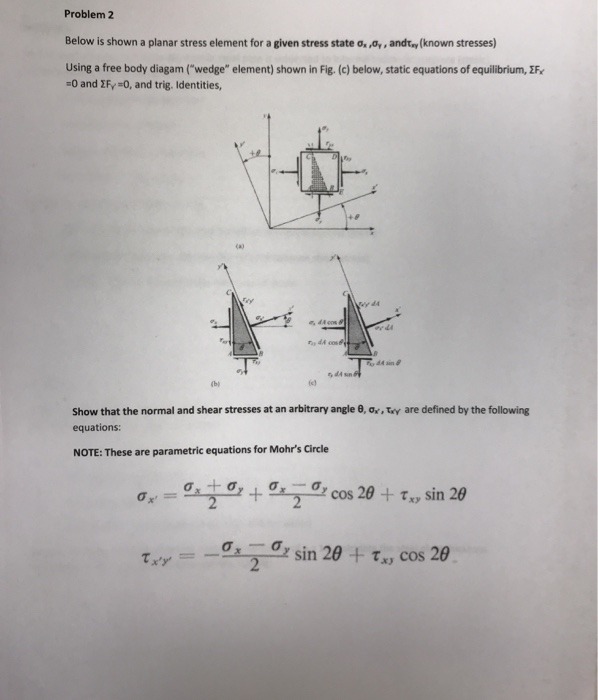Problem 2 Below is shown a planar stress element for a given stress state o.. . and known stresses) Using a free body diagam ("wedge" element) shown in Fig. (c) below, static equations of equilibrium, Fx =0 and Fy=0, and trig, Identities, Show that the normal and shear stresses at an arbitrary angle ,0x, Txy are defined by the following equations: NOTE: These are parametric equations for Mohr's Circle 0x = 6 0; + 0Os cos 2€ + sin 20...